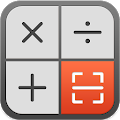# Calculator Math – Scan Math, Solve by Camera APK

## app.rsmobile.acecalculator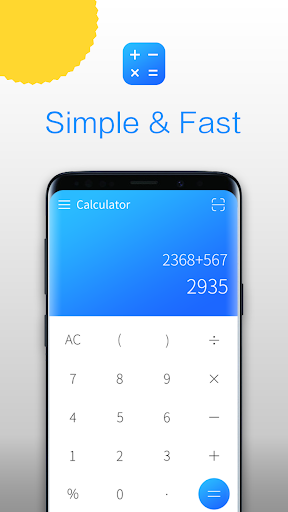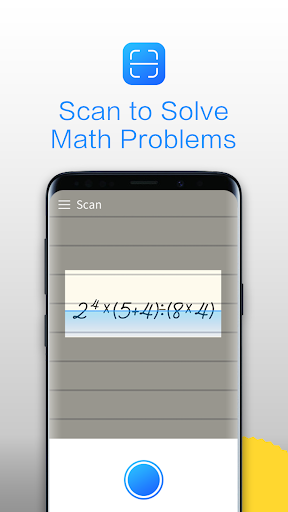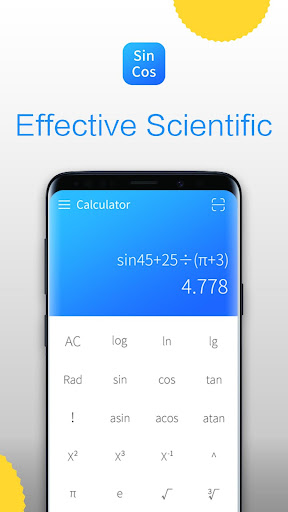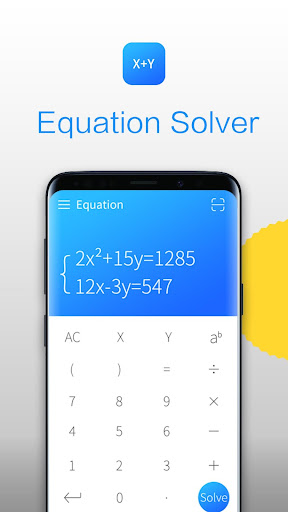📟 Scan math problems, recognize and analyze quickly, solving in few seconds. Also we enhance simple math keyboard into scientific keyboard, including sin cos tan ^ and so no. Thus, we solve formula as quickly as simple calculate.Download this app today, and Have fun with numbers.

We Calculator, a Multi Calculator APP, which can easily solve difficult math problem by entering math formula, solve math equation by entering X,Y formula, organized math problems on a writing paper. We Calculator Math lab, the perfect calculator for Android. I'm easy to use and beautifully designed to do things better than your phone or handheld calculator ever did.

We offer:
📟Free basic calculator
🔢Simple calculator keyboard: Daily Calculator, very fast for simple calculate.
🔣 Scientific Keyboard: Pull up your phone to enhance keyboard to scientific keyboard.
Multiplication Calculator: SCIENTIFIC and EQUATION， quick way to solve
Scan to get answer: Handwriting math， Solve Math by Camera
Step-by-Step Explanation： solve difficult math，make it easy to understanding.
History available：Never lose calculations

Scanning: Take photos to solve problems with typing numbers one by one, speed up and be #1 in class.
Quick way to solve math formula:
Type in your unsolve math formula, we can solve it within few secounds
Calculating History:

You can use our calculator when:
Solve Math formula, start interest in Math.
Calculate discount and sale price.

Special Feature:
· Scanning: Scan the handwriting math formula, answers can be easily get.
· Great Decoration: Unique style let you feel peace and focus on math calculate.
· Full support for Android: Effective and Handheld, we All-in-One and Effective and Handheld

Please give 5 star rating if we are doing good.